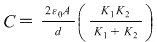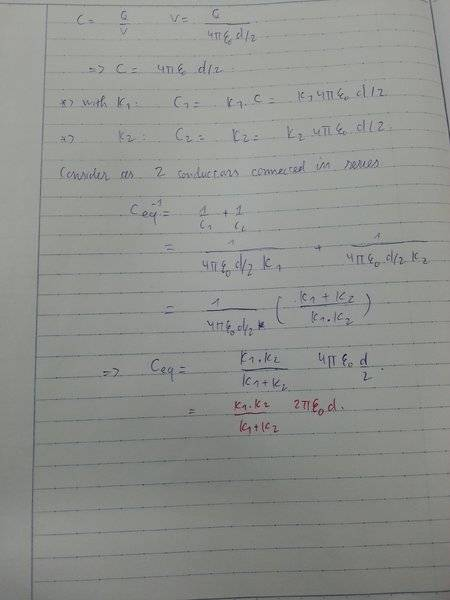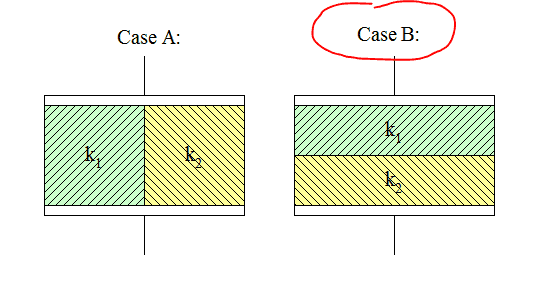# Confusion about a capacitor with 2 different dielectrics

1. The problem statement, all variables, and given/known data
A parallel-plate capacitor has the space between the plates filled with to slabs of dielectric, one with constant K1, & one constant K2. each slab has thickness d⁄2, where d is plate separation, show the capacitance is?## Homework Equations

C=Q/V
E=Q/(ε0.A)V=Q/(4πε0.r)

## The Attempt at a Solution

I consider the conductor as 2 different conductors with different slabs of the dielectric. I think that is not a bad idea to find the answer, but my result is different from the answer, maybe it is wrong somewhere. Can you guys help me? Here is my solution.#### Attachments

TSny
Homework Helper
Gold Member
Hello. Welcome to PF!

The mistake is using the equation ##V = \large \frac{Q}{4\pi\epsilon_0 r}##. This is not applicable to parallel plates.

•Hieu and Vriska
You can express V as a line integral over the E field. Try using: $$V=\int_0^d E(z)dz=\int_0^{\frac {d} {2}} E_1(z)dz +\int_{\frac {d} {2}}^{d} E_2(z)dz$$where ##E=\frac {\sigma} {K\epsilon_0}##, d is plate separation and ##\sigma## is the charge density.

Peace
Fred

•Hieu
gneill
Mentor
Hi Hieu,

Your problem statement should be more specific about the geometric arrangement of the dielectric slabs between the plates. Do they divide the space horizontally or vertically? Given the expected answer we can deduce that the arrangement is two horizontal slabs dividing the space vertically (case B below), but it might be not so obvious to a new student approaching this type of problem for the first time.#### Attachments

•Hieu
CWatters
•